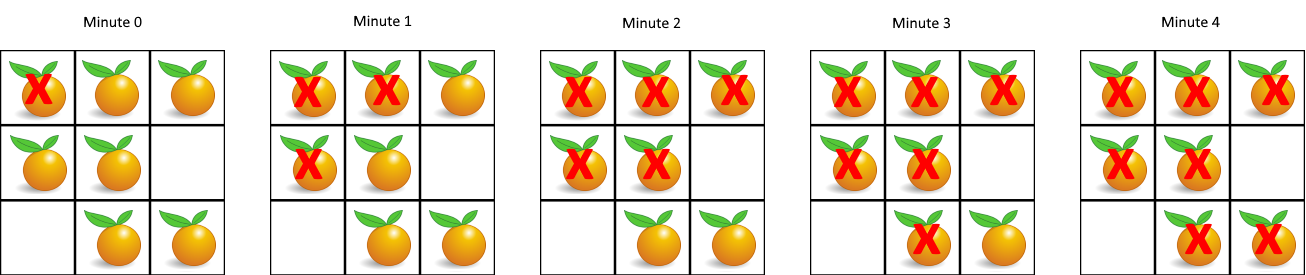# LeetCode Rotting Oranges Solution

You are given an `m x n` `grid` where each cell can have one of three values:

• `0` representing an empty cell,
• `1` representing a fresh orange, or
• `2` representing a rotten orange.

Every minute, any fresh orange that is 4-directionally adjacent to a rotten orange becomes rotten.

Return the minimum number of minutes that must elapse until no cell has a fresh orange. If this is impossible, return `-1`.

Example 1:Input: `grid = [[2,1,1],[1,1,0],[0,1,1]]`
Output: `4`

Example 2:

Input: `grid = [[2,1,1],[0,1,1],[1,0,1]]`
Output: `-1`
Explanation: The orange in the bottom left corner (row 2, column 0) is never rotten, because rotting only happens 4-directionally.

Example 3:

Input: `grid = [[0,2]]`
Output: `0`
Explanation: Since there are already no fresh oranges at minute 0, the answer is just 0.

Constraints:

• `m == grid.length`
• `n == grid[i].length`
• `1 <= m, n <= 10`
• `grid[i][j]` is `0`, `1`, or `2`.

## Solution

Since we are asked to find the when we will reach the final stage of the oranges, we must find the final stage first. Each minute, the rotten oranges will rot its neighboring oranges, thus the best way to approach this question is BFS starting with the rotten oranges, each minute we expand the breadth by 1 layer. We keep a counter of the minutes passed, and in the very end check whether all oranges are rotten.

We will follow these steps to implement this problem.

1. put all rotten oranges into a queue
2. BFS on queue to rot other oranges, `minutes` = number of levels in BFS
3. check whether there are unrotten oranges (return -1)
``````1def orangesRotting(grid: List[List[int]]) -> int:
2    m = len(grid)
3    n = len(grid)
4    minute = -1
5    rot = deque()
6    has_orange = False            # whether grid contain an orange
7    for x in range(m):
8        for y in range(n):
9            has_orange = has_orange or grid[x][y]
10            if grid[x][y] == 2:   # rotten orange
11                rot.append((x, y))
12    if not has_orange: return 0   # no orange in grid, return 0
13    while rot:          # bfs to rot oranges
14        minute += 1
15        count = len(rot)
16        for _ in range(count):
17            x, y = rot.popleft()
18            if x+1 < m and grid[x+1][y] == 1:
19                    grid[x+1][y] = 2
20                    rot.append((x+1, y))
21            if x-1 >= 0 and grid[x-1][y] == 1:
22                    grid[x-1][y] = 2
23                    rot.append((x-1, y))
24            if y+1 < n and grid[x][y+1] == 1:
25                    grid[x][y+1] = 2
26                    rot.append((x, y+1))
27            if y-1 >= 0 and grid[x][y-1] == 1:
28                    grid[x][y-1] = 2
29                    rot.append((x, y-1))
30    for x in range(m):    # check for unrotten oranges
31        for y in range(n):
32            if grid[x][y] == 1:
33                return -1
34    return minute``````